# Maths - Domain Theory

A domain is a particular type if poset.

They come from attempts to give semantics to recursivly defined functions and types.

## Example of Application to Computer Languages

 Many computer languages allow us to define functions recursively, for example 'factorial': ```factorial(n) if (n=0) return 1 otherwise return [ n × factorial(n-1) ]```

Types can also be defined recursively like this:A list could be implemented like this: L(x)=1+x•L(x) = 1+x+x²+x³... see page here

How can we be sure that these recursive definitions always return a valid result?

These recursive definitions tend to have a common form:

1. A partial function (in above case: 'if n=0, return 1').
2. If partial function is undefined then call self.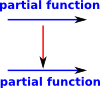In this diagram the blue arrows represent partial functions and the red arrow represents one level of recursion.So we can see in the factorial example, at each level of recursion the partial function becomes less partial. So these partial functions form a chain: P0P1P2 ...

## Denotional Semantics

We use double square brackets '[[' and ']]' to represent semantic braces. These surround some expression to represent the result of that expression.

As an example imagine that we have a system for calculating the results of a numerical calculation. The syntax is defined inductively as follows:

term ::= number | term + term | term - term | term * term | term / term

where

number ::= 0,1,2,3,4,5,6,7...

We then use the semantic braces to represent the semantics. In this case every term evaluates to a number:

[[term]] = number

The point of denotional semantics is to define how this is done, so we break this down.

For example '3' evaluates to 3:

[] = 3

In fact any number literal evaluates to that number:

[[number]] = number

Here we use 'number' to represent any number, we don't want to do that sort of thing too often or denotional semantics wouldn't add anything at all, here we have to do this otherwise we would have to define every number.

we define the operations like this:

[[a+b]] = [a]+[b]

Unfortunately we have used the '+' symbol for two different things here. On the left it is just a syntactical operation, on the right it is the mathematical operation of add.

This is a very simple example, we now want to go on to show how this can define recursive functions, but first we compare with similar looking approaches.

#### Logic

Denotional semantics seems similar to logic, for instance there are rules like modus ponens:

from A and A -> B infer B

However not all logic rules lead to a simplification

#### Lambda Calculus

Lambda calculus has variables which we can replace with the actual values during evaluation.

## Partial Functions

When we discussed mappings and functions, on the page here, we will mostly assumed that every element in the domain mapped to an element in the domain, however this is not the case for partial functions.

Examples of partial functions, in the real numbers, are:

• x1/x (not defined for x=0)
• xsqrt(x) (not defined for negative numbers)

This is also interesting in computing where a function may represent a computation. Where this computation is very complicated it may not be possible to be sure that every input to the function will produce a valid result.

 On the right is a representation of a partial function as a graph. The function 'f' is defined for inputs 'a' and 'c' but not 'b' and 'd'.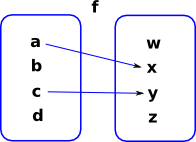Here is another partial function 'g'. This is related to the function 'f' above because, if defines everything that 'f' defines but it also defines a result for 'b'. This situation where function 'g' is defined as-well-as-or-better than 'f' is represented symbolically as: fgWe can define a whole 'chain' of partial functions. efg Below 'f' we have a partial function which specifies an output for a single input, we can build up results upto a compete function.At the bottom of this chain we have a partial function which does not map any of its elements. We denote this by the 'bottom' symbol: ''efg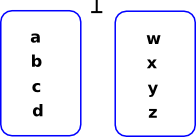A partial function can be made into a total function in various ways:

• We could choose a subset of the domain which is mapped to the codomain
• We could add an element to the codomain to represent 'undefined' then all undefined values can be mapped to this.

The first option may not be possible because we may not know which values have valid results or such a subset may be difficult to define.

### Partial Function in Category TheoryA partial function from A to B is defined by the span shown on the left. D →B is a total function. D →A is an injective function. Note: an arrow from A to B is not shown since arrows represent total functions.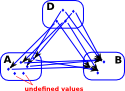## Domains

A domain is a poset where:

• Various types of joins exist (a kind of co-completeness property).
• The element(bottom element) exists.

Examples of such domains are ωcpos and dcpos.

### Applications

This has been used in defining the semantics of programming languages (denotational semantics). Particularly recursively defined functions.

### Recursively Defined Functions

#### Example Factorial

For example we could define factorial like this:

`fact = λ n . if n=0 return 1 else return n*fact(n-1) `

The recursion comes because the function is used in its own definition.

 This generates a linear sequence of values where each one depends on the previous one.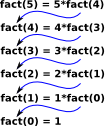Alternatively we could draw it as a sequence of ordered input-output pairs like this:The denotion for a recursive function is a set of ordered pairs, in this case: We could think of this chain as a set of points on a graph. In this case: graph(factorial) {[] [1 1] [2 2] [3 6] [4 24]...[n !n]}

The least upper bound of this chain is the factorial function.

So a recursively defined function can be understood in terms of a family of non-recursive denotions.

### Fixed Point Property

Above we defined the factorial like this:

`fact = λ n . if n=0 return 1 else return n*fact(n-1) `

So 'fact':N->N is the factorial function from N to N, given a number it will return the factorial of it.

But we could treat this like an equation where 'fact' is the unknown.

`F = λ fact . λ n . if n=0 return 1 else return n*fact(n-1) `

Now we take 'graph' to represent a graph like inputs on one axis and outputs on another and the relationship shown by a subset of the plane. In this case graph is:

{[] [1 1] [2 2] [3 6] [4 24]...[n !n]}

For fixpoint we have:

graph(F(fact)) = graph(fact)

F(fact) = fact

where:

• F: (N+ -> N+) -> (N+ -> N+) is a 'functional' (a function from one function to another).
• fact:N->N is the factorial function from N to N.
• N is natural number
• N+ is extended natural number to include.

So each level in the recursion only defines part of the function:

• First level: {[] [0 1]} only defines output for input of 0.
• Second level: {[] [0 1] [1 1]} only defines output for input of 0 or 1.
• Third level: {[] [0 1] [1 1] [2 2]} only defines output for input of 0,1 or 2.
• Forth level: {[] [0 1] [1 1] [2 2] [3 6]} only defines output for input of 0,1,2 or 3.
• ...

More about fixpoints on the page here.

### LUB (Least Upper Bound) Lemma

Every chain fi has a least upper bound.

#### Example

This example illustrates a least upper bound although the function is not defined in a recursive way.

We define a chain of functions fi: f1,f2,f3...
given by: if x < i then x! else.

So fifi+1

The least upper bound is the factorial function: x x!### Functionals

A 'functional' maps a set of functions (with a given signature) back to itself.

Possible properties that functionals may have: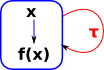#### Monotonicity

if fg implies τ[f]τ[g]

for all f,g

#### Continuity

τ[lub{fi}]=lub{τ[fi]}

where:

• τ = functional
• lub = least upper bound
• [ ] = set of functions
• { } = chain of functions

#### Data Types

We can make existing datatypes (initial algebras) into denotions as an extension.

We add bottom () to datatypes to represent unknown value. This allows the functions to be partial. So:

• a->means the value is undefined for argument 'a'.
•-> a means we are giving a value to 'a'.

Sometimes if we are extending a domain 'D' then we denote the extended domain as 'D+'

 For example boolean values: Note: for all these extended domains there is an identity arrow from every element to itself, but I have not shown that on the following diagrams to avoid clutter.Or Natural Numbers: Here we have two levels of ordering: The ordering of the natural numbers: ≤ The information ordering:Example in reals:

inverse = λ x . 1/x

so, for inverse, we have 0->#### Combining

Bottom Information Ordering

Product

We can take the
Cartesian product
of two domains
A and B.

If:

• bottom of A isA and
• bottom of B isB then:
• bottom of A×B is <A,B>

If:

• a1a2 and
• b1b2 then:
• <a1,b1><a2,b2>

Sum
disjoint (except) union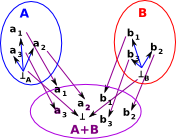A andB both map toin A+B

Exponent
(function)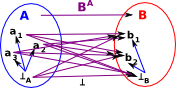For f,gHom(A,B)

fg if f(x)Bg(x) for all xAOn these diagrams the purple arrows represent the underlying relation and the blue arrows represent the 'less or equally defined' relationship.

Types of product for graphs (Cartesian and tensor) are discussed on the page here.

The product of domains starts to show how we get more complexity and more layers in domains.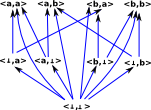#### Natural Extension

If we combine all nodes with at least oneinto a single node calledthen we get back to the simple two layer structure.

A morphism where any nodes with acomponent map to a singlenode is called a 'natural extension'. This makes the function monotonic.The elements of the exponent BA are all the possible arrows from A to B.

• Those arrows which don't involverepresent the mappings between A and B.
• Those arrows which end atB represent undefined mappings so we can represent partial mappings.

### Meaning of these Elements in Denotational Semantics

#### Arrow

If we have an arrow such as A->B the following notation: AB is often used, even when we don't necessarily mean that A is a subset of B. However to make the distinction clearer, here we will use a squared off form of this notation: AB. This tends to mean that:

• A is less defined than B
• or A approximates to B

The relation AB is:

• reflexive AA
• transitive AB and BC implies AC
• anti-symmetric AB and BA implies A=B

#### Bottom

The denotion '' often represents a computation that is undefined or fails to terminate.

## Extended Domain

So far the extended domain has only 2 levels, that is, there is a direct arrow fromto every other element. When we define structures recursively then we get more structure, we get a whole chain where each element is more defined.

## Partially Defined Functions

In the S=set {0,1,2} we define a minus '-' function as follows:

Since we don't have negative numbers some of the operations will be undefined.

0 1 2
0 0 1 2
1 undefined 0 1
2 undefined undefined 0
We can make the function fully defined we can extend S to S+, we do this by adding.0 1 200 1 2
10 1
20

### Domain Theory### As Partially Ordered Set

here we look at a poset represented as a directed graph (like Hasse diagram).

 If we have a finite directed graph and we repeatedly follow the arrows then we will eventually get to either: A multiple node loop. A single node loop. A dead end.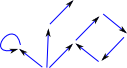If we have a meet-semilattice then we will always end up eventually at the maximal element. If we have a join-semilattice and we go in the reverse direction to the arrows, then we will always end up eventually at the minimal element.Above diagram redrawn here as a sequence of maps. Each row represents a map D -> D.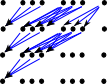### Definitions

 Directed Set (or directed preorder) set with reflective and transitive binary relation (partially ordered sets need not be directed) dcpos Directed co-complete partial order. ωcpos ω-chain co-complete partial order. A poset in which every ω-chain has a supremum chain For every x,yC then x≤y or x≥y Sometimes chain is used as another name for 'totally ordered set'. It can also be used for a totally ordered subset of a poset. ω-chain Its elements can be indexed by the natural numbers. anti-chain For every x,yC then x=y ( x≤y and x≥y) complete existence of meets for subsets co-complete existence of joins for subsets

### Functions between Preordered Sets

A function between preordered sets preserves structure, in this case the order, this is also known as a monotone function.

 A function between preordered sets is: monotone if x->y implies f(x)->f(y). antitone if x->y implies f(y)->f(x).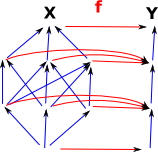### Algebraic Closure System

Algebraic closure systems are systems of subalgebras of finitary algebras (sets with a family of finitary operations).

Given:

• Set 'A'
• Set 'C' of subsets of 'A'

Closed under the intersection of arbitrary subcollections is called a closure system over 'A'.

If 'C' is also closed under the union of subcollections that are upward directed then it is an algebraic closure system.

An algebraic closure system forms an algebraic lattice under the set theoretic inclusion order.

 metadata block see also: other sites: Correspondence about this page Book Shop - Further reading. Where I can, I have put links to Amazon for books that are relevant to the subject, click on the appropriate country flag to get more details of the book or to buy it from them. Specific to this page here:

This site may have errors. Don't use for critical systems.

Copyright (c) 1998-2021 Martin John Baker - All rights reserved - privacy policy.